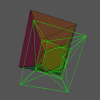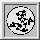Up: Qhull manual: contents
To: ProgramsOptionsOutputFormatsGeomviewPrintQhullPrecisionTraceFunctions (local)
To: synopsis • input • outputs • controls • graphics • notes • conventions • options

#qvoronoi Qu -- furthest-site Voronoi diagram

The furthest-site Voronoi diagram is the furthest-neighbor map for a set of points. Each region contains those points that are further from one input site than any other input site. See the survey article by Aurenhammer ['91] and the brief introduction by O'Rourke ['94]. The furthest-site Voronoi diagram is the dual of the furthest-site Delaunay triangulation.

Example: rbox 10 D2 | qvoronoi Qu s o TO result
Compute the 2-d, furthest-site Voronoi diagram of 10 random points. Write a summary to the console and the Voronoi regions and vertices to 'result'. The first vertex of the result indicates unbounded regions. Almost all regions are unbounded.
Example: rbox r y c G1 D2 | qvoronoi Qu s Fn TO result
Compute the 2-d furthest-site Voronoi diagram of a square and a small triangle. Write a summary to the console and the Voronoi vertices for each input site to 'result'. The origin is the only furthest-site Voronoi vertex. The negative indices indicate vertices-at-infinity.

Qhull computes the furthest-site Voronoi diagram via the furthest-site Delaunay triangulation. Each furthest-site Voronoi vertex is the circumcenter of an upper facet of the Delaunay triangulation. Each furthest-site Voronoi region corresponds to a vertex of the Delaunay triangulation (i.e., an input site).

See Qhull FAQ (local) - Delaunay and Voronoi diagram questions.

The 'qvonoroi' program is equivalent to 'qhull v Qbb'. It disables the following Qhull options: d n m v H U Qb QB Qc Qf Qg Qi Qm Qr Qv Qx TR E V Fa FA FC Fp FS Ft FV Gt Q0,etc.

### »furthest-site qvoronoi synopsis

See qvoronoi synopsis. The same program is used for both constructions. Use option 'Qu' for furthest-site Voronoi diagrams.

### »furthest-site qvoronoi input

The input data on stdin consists of:

• dimension
• number of points
• point coordinates

Use I/O redirection (e.g., qvoronoi Qu < data.txt), a pipe (e.g., rbox 10 | qvoronoi Qu), or the 'TI' option (e.g., qvoronoi TI data.txt Qu).

For example, this is a square containing four random points. Its furthest-site Voronoi diagram has on vertex and four unbounded, separating hyperplanes (i.e., the coordinate axes)

rbox c 4 D2 > data
```2 RBOX c 4 D2
8
-0.4999921736307369 -0.3684622117955817
0.2556053225468894 -0.0413498678629751
0.0327672376602583 -0.2810408135699488
-0.452955383763607 0.17886471718444
-0.5   -0.5
-0.5    0.5
0.5   -0.5
0.5    0.5
```

qvoronoi Qu s Fo < data

```
Furthest-site Voronoi vertices by the convex hull of 8 points in 3-d:

Number of Voronoi regions: 8
Number of Voronoi vertices: 1
Number of non-simplicial Voronoi vertices: 1

Statistics for: RBOX c 4 D2 | QVORONOI Qu s Fo

Number of points processed: 8
Number of hyperplanes created: 20
Number of facets in hull: 11
Number of distance tests for qhull: 34
Number of merged facets: 1
Number of distance tests for merging: 107
CPU seconds to compute hull (after input):  0

4
5 4 5      0      1      0
5 4 6      1      0      0
5 5 7      1      0      0
5 6 7      0      1      0
```

### »furthest-site qvoronoi outputs

These options control the output of furthest-site Voronoi diagrams.

furthest-site Voronoi vertices
p
print the coordinates of the furthest-site Voronoi vertices. The first line is the dimension. The second line is the number of vertices. Each remaining line is a furthest-site Voronoi vertex. The points-in-square example has one furthest-site Voronoi vertex at the origin.
Fn
list the neighboring furthest-site Voronoi vertices for each furthest-site Voronoi vertex. The first line is the number of Voronoi vertices. Each remaining line starts with the number of neighboring vertices. Negative indices (e.g., -1) indicate vertices outside of the Voronoi diagram. In the points-in-square example, the Voronoi vertex at the origin has four neighbors-at-infinity.
FN
list the furthest-site Voronoi vertices for each furthest-site Voronoi region. The first line is the number of Voronoi regions. Each remaining line starts with the number of Voronoi vertices. Negative indices (e.g., -1) indicate vertices outside of the Voronoi diagram. In the points-in-square example, all regions share the Voronoi vertex at the origin.

furthest-site Voronoi regions
o
print the furthest-site Voronoi regions in OFF format. The first line is the dimension. The second line is the number of vertices, the number of input sites, and "1". The third line represents the vertex-at-infinity. Its coordinates are "-10.101". The next lines are the coordinates of the furthest-site Voronoi vertices. Each remaining line starts with the number of Voronoi vertices in a Voronoi region. In 2-d, the vertices are listed in adjacency order (unoriented). In 3-d and higher, the vertices are listed in numeric order. In the points-in-square example, each unbounded region includes the Voronoi vertex at the origin. Lines consisting of 0 indicate interior input sites.
Fi
print separating hyperplanes for inner, bounded furthest-site Voronoi regions. The first number is the number of separating hyperplanes. Each remaining line starts with 3+dim. The next two numbers are adjacent input sites. The next dim numbers are the coefficients of the separating hyperplane. The last number is its offset. The are no bounded, separating hyperplanes for the points-in-square example.
Fo
print separating hyperplanes for outer, unbounded furthest-site Voronoi regions. The first number is the number of separating hyperplanes. Each remaining line starts with 3+dim. The next two numbers are adjacent input sites on the convex hull. The next dim numbers are the coefficients of the separating hyperplane. The last number is its offset. The points-in-square example has four unbounded, separating hyperplanes.

Input sites
Fv
list ridges of furthest-site Voronoi vertices for pairs of input sites. The first line is the number of ridges. Each remaining line starts with two plus the number of Voronoi vertices in the ridge. The next two numbers are two adjacent input sites. The remaining numbers list the Voronoi vertices. As with option 'o', a 0 indicates the vertex-at-infinity and an unbounded, separating hyperplane. The perpendicular bisector (separating hyperplane) of the input sites is a flat through these vertices. In the points-in-square example, the ridge for each edge of the square is unbounded.

General
s
print summary of the furthest-site Voronoi diagram. Use 'Fs' for numeric data.
i
list input sites for each furthest-site Delaunay region. Use option 'Pp' to avoid the warning. The first line is the number of regions. The remaining lines list the input sites for each region. The regions are oriented. In the points-in-square example, the square region has four input sites. In 3-d and higher, report cospherical sites by adding extra points.
G
Geomview output for 2-d furthest-site Voronoi diagrams.

### »furthest-site qvoronoi controls

Qu
must be used.
QRn
randomly rotate the input with a random seed of n. If n=0, the seed is the time. If n=-1, use time for the random seed, but do not rotate the input.
QVn
select furthest-site Voronoi vertices for input site n
Tv
verify result
TI file
input data from file. The filename may not use spaces or quotes.
TO file
output results to file. Use single quotes if the filename contains spaces (e.g., TO 'file with spaces.txt'
TFn
report progress after constructing n facets
PDk:1
include upper and lower facets in the output. Set k to the last dimension (e.g., 'PD2:1' for 2-d inputs).
f
facet dump. Print the data structure for each facet (i.e., furthest-site Voronoi vertex).

### »furthest-site qvoronoi graphics

In 2-d, Geomview output ('G') displays a furthest-site Voronoi diagram with extra edges to close the unbounded furthest-site Voronoi regions. All regions will be unbounded. Since the points-in-box example has only one furthest-site Voronoi vertex, the Geomview output is one point.

See the Delaunay and Voronoi examples for a 2-d example. Turn off normalization (on Geomview's 'obscure' menu) when comparing the furthest-site Voronoi diagram with the corresponding Voronoi diagram.

### »furthest-site qvoronoi notes

See Voronoi notes.

### »furthest-site qvoronoi conventions

The following terminology is used for furthest-site Voronoi diagrams in Qhull. The underlying structure is a furthest-site Delaunay triangulation from a convex hull in one higher dimension. Upper facets of the Delaunay triangulation correspond to vertices of the furthest-site Voronoi diagram. Vertices of the furthest-site Delaunay triangulation correspond to input sites. They also define regions of the furthest-site Voronoi diagram. All vertices are extreme points of the input sites. See qconvex conventions, furthest-site delaunay conventions, and Qhull's data structures.

• input site - a point in the input (one dimension lower than a point on the convex hull)
• point - a point has d+1 coordinates. The last coordinate is the sum of the squares of the input site's coordinates
• vertex - a point on the upper facets of the paraboloid. It corresponds to a unique input site.
• furthest-site Delaunay facet - an upper facet of the paraboloid. The last coefficient of its normal is clearly positive.
• furthest-site Voronoi vertex - the circumcenter of a furthest-site Delaunay facet
• furthest-site Voronoi region - the region of Euclidean space further from an input site than any other input site. Qhull lists the furthest-site Voronoi vertices that define each furthest-site Voronoi region.
• furthest-site Voronoi diagram - the graph of the furthest-site Voronoi regions with the ridges (edges) between the regions.
• infinity vertex - the Voronoi vertex for unbounded furthest-site Voronoi regions in 'o' output format. Its coordinates are -10.101.
• good facet - an furthest-site Voronoi vertex with optional restrictions by 'QVn', etc.

### »furthest-site qvoronoi options

See qvoronoi options. The same program is used for both constructions. Use option 'Qu' for furthest-site Voronoi diagrams.The Geometry Center Home Page